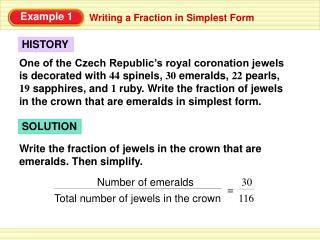DownloadDownload PresentationExample 1

# Example 1

Download Presentation## Example 1

- - - - - - - - - - - - - - - - - - - - - - - - - - - E N D - - - - - - - - - - - - - - - - - - - - - - - - - - -
##### Presentation Transcript

1. SOLUTION Write the fraction of jewels in the crown that are emeralds. Then simplify. Number of emeralds 30 = Total number of jewels in the crown 116 Example 1 Writing a Fraction in Simplest Form HISTORY One of the Czech Republic’s royal coronation jewels is decorated with 44 spinels, 30 emeralds, 22 pearls, 19 sapphires, and 1 ruby. Write the fraction of jewels in the crown that are emeralds in simplest form.

2. 30 2 • 3 5 116 22 • 29 • = = 30 30 ÷ 2 Divide numerator and denominator by GCF. = 116 116 ÷ 2 15 = Simplify. 58 Example 1 Writing a Fraction in Simplest Form METHOD 1 Find and use the GCF of 30 and 116. The GCF of 30 and 116 is 2.

3. 30 2 • 3 5 • Write prime factorizations. = 116 2 • 2 • 29 1 2 • 3 5 • 15 Divide out common factor and simplify. = = • 2 • 29 2 1 58 ANSWER 15 The fraction of jewels that are emeralds is . 58 Example 1 Writing a Fraction in Simplest Form MEHTOD 2 Use prime factorization.

4. SOLUTION Write each fraction in simplest form. 3 is in simplest form. 8 18 18 ÷ 6 3 = = 48 48 ÷ 6 8 ANSWER The fractions have the same simplest form, so they are equivalent. Example 2 Identifying Equivalent Fractions 3 18 Tell whether the fractions and are equivalent. 8 48

5. SOLUTION Multiply or divide the numerator and denominator by the same nonzero number. 4 4  3 12 Multiply numerator and denominator by 3. = = 10 10  3 30 4 4 ÷ 2 2 Divide numerator and denominator by 2, a common factor of 4 and 10. = = 10 10 ÷ 2 5 ANSWER 12 2 4 The fractions and are equivalent to . 30 5 10 Example 3 Writing Equivalent Fractions 4 Write two fractions that are equivalent to . 10

6. Guided Practice 1 ANSWER 5 7 ANSWER 25 9 ANSWER 25 4 ANSWER 25 for Examples 1, 2, and 3 Of your 25 classmates, 5 prefer apples, 7 prefer oranges, and 9 prefer bananas. Write the fraction of your classmates who prefer the given fruit in simplest form. 1. Apple 2. Orange 3. Banana 4. Other

7. Guided Practice equivalent ANSWER 10 3 , 6. not equivalent ANSWER 21 7 4 24 , 7. not equivalent ANSWER 6 30 3 , 8. equivalent ANSWER 15 7 35 for Examples 1, 2, and 3 Tell whether the fractions are equivalent. 1 4 5. , 6 24

8. Guided Practice 1 16 , ANSWER 2 32 18 3 9 , ANSWER 10. 30 5 15 7 5 42 20 10 , ANSWER 11. 6 8 24 48 12 21 , ANSWER 12. 24 for Examples 1, 2, and 3 Write two fractions that are equivalent to the given fraction. 8 9. 16